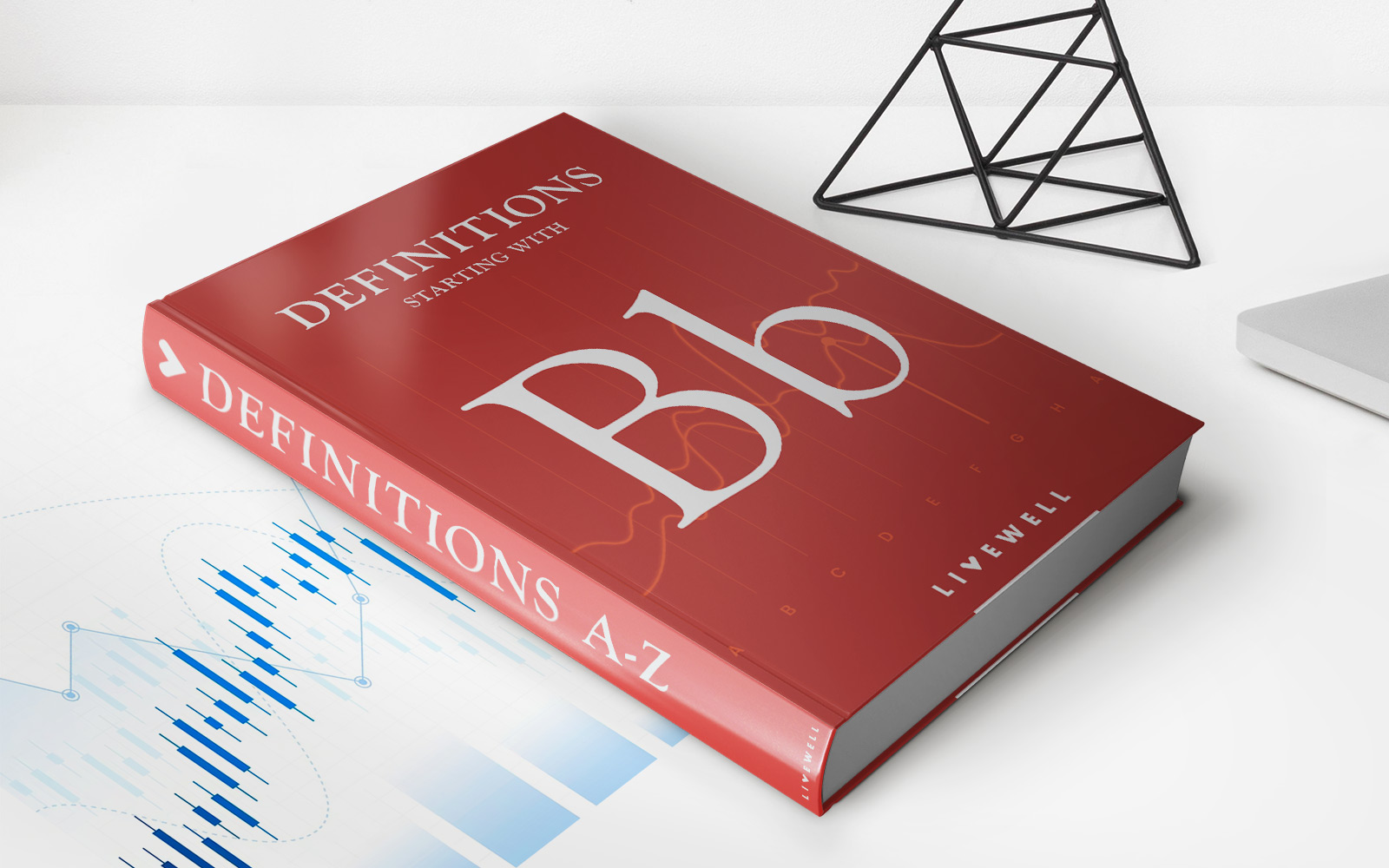Home>Finance>Bond Valuation: Calculation, Definition, Formula, And ExampleFinance

# Bond Valuation: Calculation, Definition, Formula, And Example

Learn how bond valuation works in finance, including the calculation, definition, formula, and real-life examples. Explore the fundamentals of valuing bonds and enhance your financial knowledge.

## Bond Valuation: Calculation, Definition, Formula, and Example

When it comes to investing in the financial market, understanding various financial instruments is crucial. One such tool that investors often come across is bonds. If you’re looking to invest in bonds or simply expand your financial knowledge, it’s essential to have a comprehensive understanding of bond valuation. In this article, we’ll cover what bond valuation is, its calculation methods, formulas, and provide an example to help you grasp this concept.

## Key Takeaways:

• Bond valuation is the process of determining the fair value of a bond. It helps investors analyze the potential return and risk associated with investing in a particular bond.
• The most common bond valuation methods are present value and yield to maturity.

## What is Bond Valuation?

Bond valuation is the process of calculating the fair value of a bond, which represents the present value of its future cash flows. By assessing the value of a bond, investors can determine whether it is overpriced or underpriced, helping them make informed investment decisions.

Bond valuation takes into account aspects such as the bond’s face value, coupon rate, maturity date, and yield to maturity (YTM). It considers the time value of money, which means that future cash flows are discounted back to their present value.

## Calculating Bond Value

Two commonly used methods for bond valuation are present value and yield to maturity.

1. Present Value Method:

This method calculates the present value of a bond’s future cash flows by discounting them back to today’s value using an appropriate discount rate. The formula for calculating the present value of a bond is:

Bond Value = (Coupon Payment / (1 + YTM)^1) + (Coupon Payment / (1 + YTM)^2) + … + (Coupon Payment + Face Value / (1 + YTM)^n)

Where:

• Coupon Payment is the periodic interest payment made by the bond issuer to the bondholder.
• YTM is the yield to maturity, representing the rate of return the bondholder would earn if they held the bond until maturity.
• n is the number of periods until maturity.

2. Yield to Maturity (YTM) Method:

This method determines the yield (rate of return) an investor would earn if they held the bond until maturity. To calculate YTM, you need to solve the bond valuation equation using a trial-and-error approach or financial calculator. By determining the YTM, investors can assess the attractiveness of the bond and compare it with other investment options.

## Example of Bond Valuation

Let’s consider an example to better understand bond valuation:

Company XYZ issues a bond with a face value of \$1,000, a coupon rate of 5%, and a maturity of 5 years. The prevailing interest rate in the market for similar bonds is 4.5%. Using the present value method, we can calculate the bond’s value:

Bond Value = (50 / (1 + 0.045)^1) + (50 / (1 + 0.045)^2) + (50 / (1 + 0.045)^3) + (50 / (1 + 0.045)^4) + (1,050 / (1 + 0.045)^5)

Bond Value = \$1,066.08

In this example, the bond’s value is higher than its face value, indicating that the bond is priced at a premium. Investors need to evaluate whether this premium is justified based on their return expectations and the associated risks.

By understanding bond valuation, investors can assess the attractiveness of a bond investment and make informed decisions. Remember to consider the bond’s features, such as the coupon rate, face value, maturity, and prevailing interest rates, when calculating its present value or yield to maturity. Stay vigilant and analyze the potential risks and returns before making any investment choices.

Hopefully, this article has helped expand your understanding of bond valuation and its calculation methods. If you have any further questions or need professional advice on bond investments, it’s always best to consult a financial advisor.

### Related Post

• https://livewell.com/finance/bond-valuation-calculation-definition-formula-and-example/